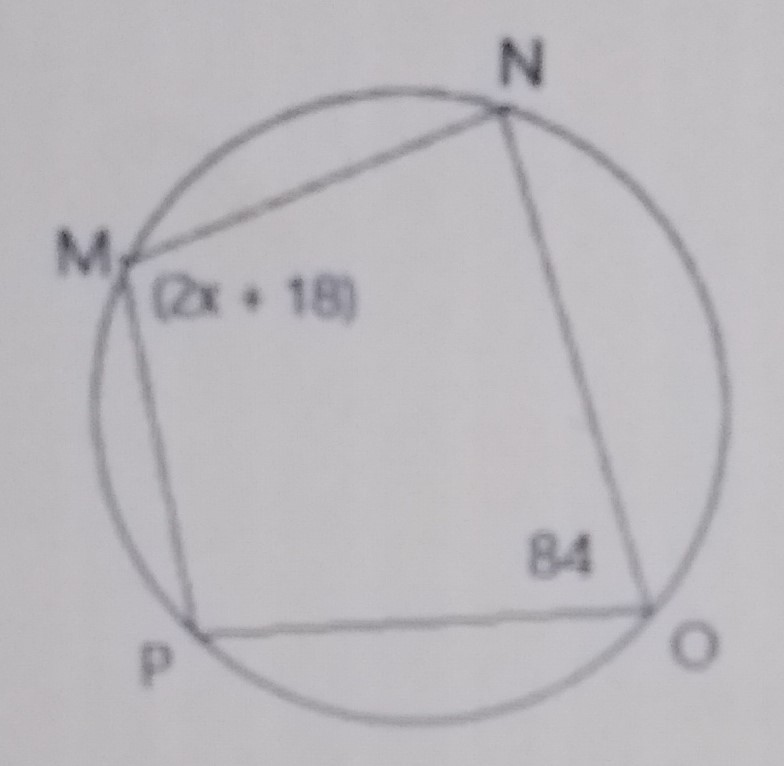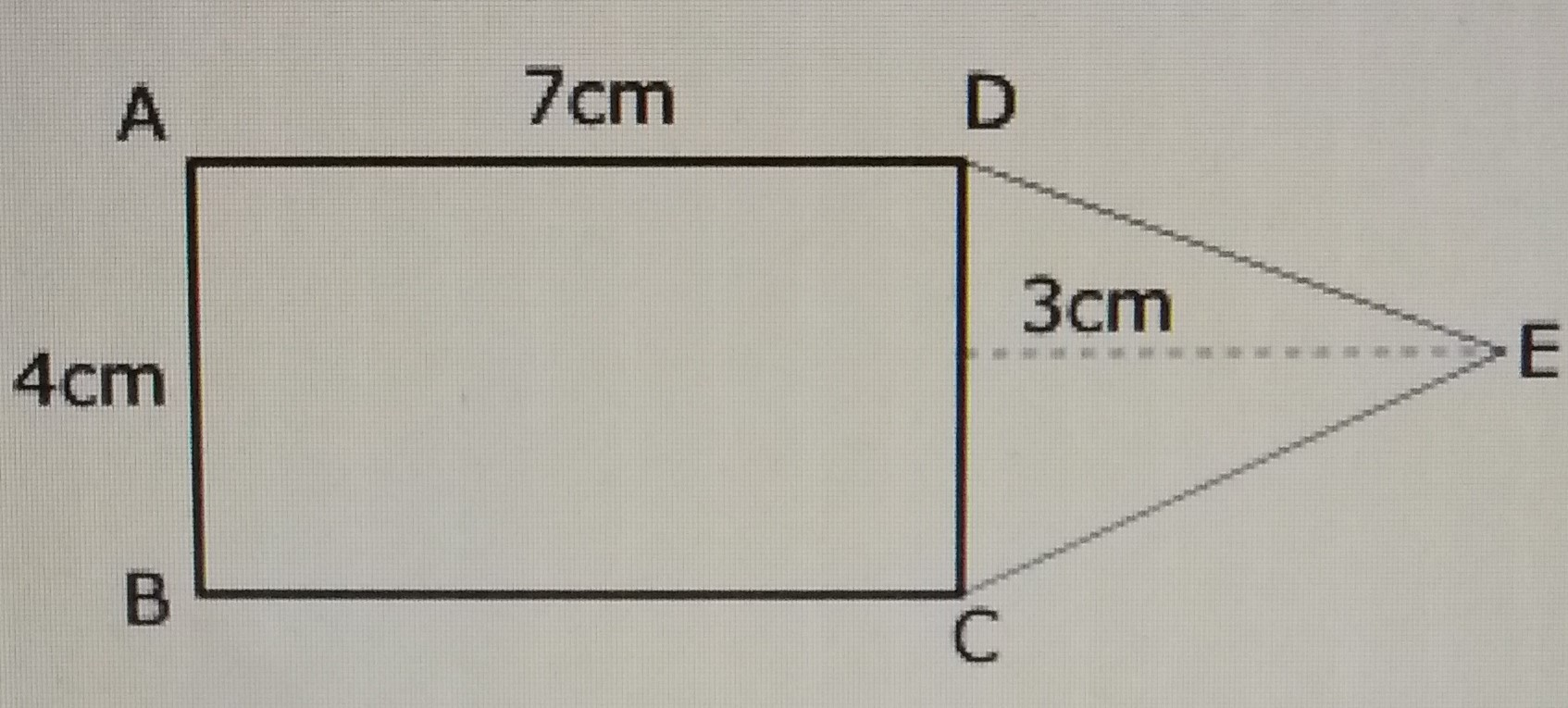# Mathematics 2019 Past Questions | JAMB

Study the following Mathematics past questions and answers for JAMBWAEC  NECO and Post-JAMB. Get prepared with official past questions and answers for upcoming examinations.

11. If a fair coin is tossed 3 times, what is the probability of getting at least two heads?

• A.$$\frac{2}{3}$$
• B.$$\frac{4}{5}$$
• C. $$\frac{2}{5}$$
• D. $$\frac{}{2}$$

12. In how many ways can the word MATHEMATICIAN be arranged?

• A. 6,794,800 ways
• B. 2,664,910 ways
• C. 6,227,020,800 ways
• D. 129,729,600 ways

13. Given matrix M = $$\begin{vmatrix} -2 ~~~ 0 ~~~ 4 \\ 0 ~~~ -1 ~~~ 6 \\ 5 ~~~ 6 ~~~ 3 \end{vmatrix}$$, find $$M^{T} + 2M$$

• A.
$$\begin{vmatrix} -4 & 2 & 1\\ 6 & 0 & 5 \\ 0 & 6 & 2 \end{vmatrix}$$
• B.
$$\begin{vmatrix} -6 & 0 & 13\\ 0 & -3 & 18 \\ 14 & 18 & 9 \end{vmatrix}$$
• C.
$$\begin{vmatrix} 5 & 2 & 6 \\ 0 & 1 & 1\\ 3 & 4 & -7 \end{vmatrix}$$
• D.
$$\begin{vmatrix} -4 & 0 & 8 \\ 0 & -2 & -16 \\ 10 & 12 & 6 \end{vmatrix}$$

14.

 Score (x) 0 1 2 3 4 5 6 Freq (f) 5 7 3 7 11 6 7

Find the mean of the data.

• A. 3.26
• B. 4.91
• C. 6.57
• D. 3.0

15.

 Score (x) 0 1 2 3 4 5 6 Freq (f) 5 7 3 7 11 6 7

Find the variance

• A. 42
• B. 20
• C. 70
• D. 54

16. The locus of a point which moves so that it is equidistant from two intersecting straight lines is the

• A. bisector of the two lines
• B. line parallel to the two lines
• C. angle bisector of the two lines
• D. perpendicular bisector of the two lines

17.From the cyclic quadrilateral MNOP above, find the value of x.

• A. 16°
• B. 25°
• C. 42°
• D. 39°

18.  If 4sin2x3=0$4{\mathrm{sin}}^{2}x-3=0$, find the value of x, when 0° $\le$ x $\le$ 90°

• A. 90°
• B. 45°
• C. 60°
• D. 30°

19.In the figure above, |CD| is the base of the triangle CDE. Find the area of the figure to the nearest whole number.

• A. 56 cm2${}^{2}$
• B. 24 cm2${}^{2}$
• C. 42 cm2${}^{2}$
• D. 34 cm2

20. The marks scored by 30 students in a Mathematics test are recorded in the table below:

 Scores (Mark) 0 1 2 3 4 5 No of students 4 3 7 8 6 2

What is the total number of marks scored by the children?

• A. 82
• B. 15
• C. 63
• D. 75

Scroll to Top